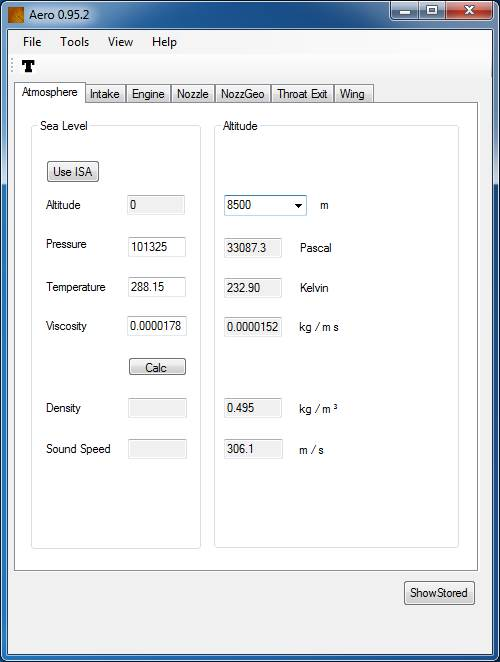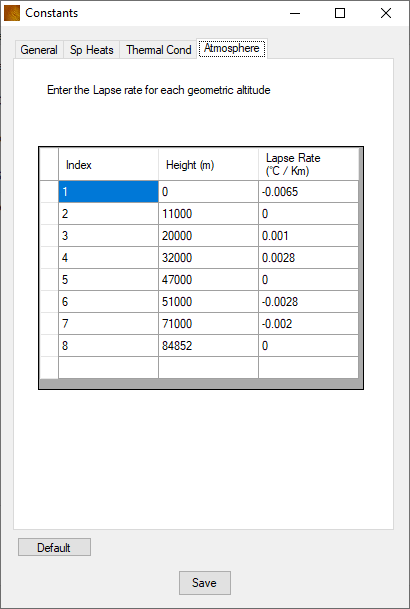CaidedTab 1 - Atmosphere

On the left hand pane, the Altitude is set to zero.
Sea level conditions can therefore be calculated.

Enter a Pressure , Temperature and Viscosity and the density  can be calculated using the Gas Equation of State for perfect gases.

Use the ISA button to set these back to defaults

The speed of sound is calculated from the following relation

Use the top menu , Tools - Options and check the Fluid Mechanics check box to display the Prandtl Number

The Gas Constant R can be changed in Tools - Constants under the General tab. It is set to 287.06 J / kg K by default.

Press the Calc button to perform the calculationWithin the right hand pane, the Altitude can be changed with calculated variables changing automatically.

The slider can also be dragged up or down to alter the altitude.

These calculated values are used by the nozzle calculation when using an NPR.

If a sea level conditions are required leave this value as zero.

In Tools - Constants, under the Atmosphere tab the lapse rate can be changed for each of the 7 levels, click save to commit these values.